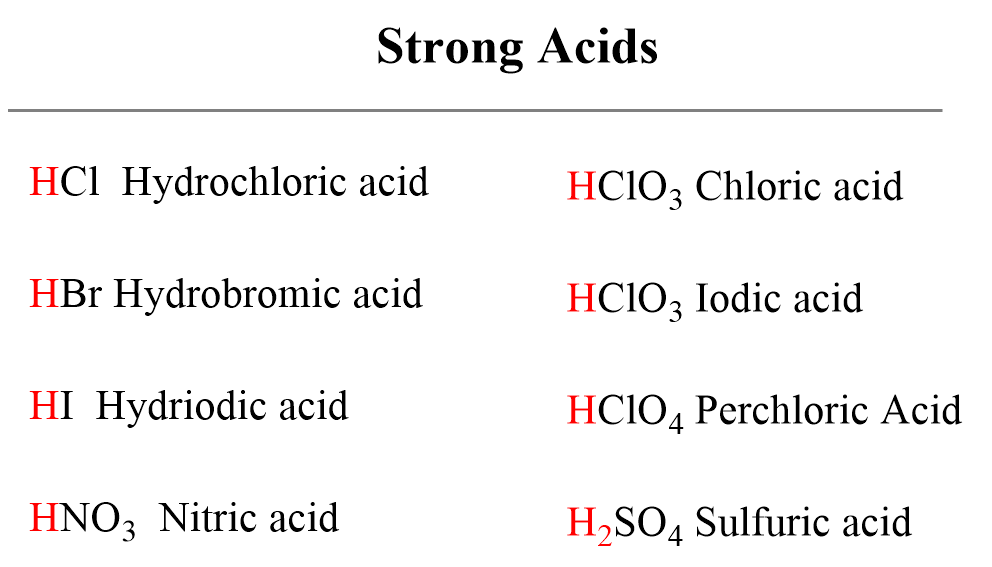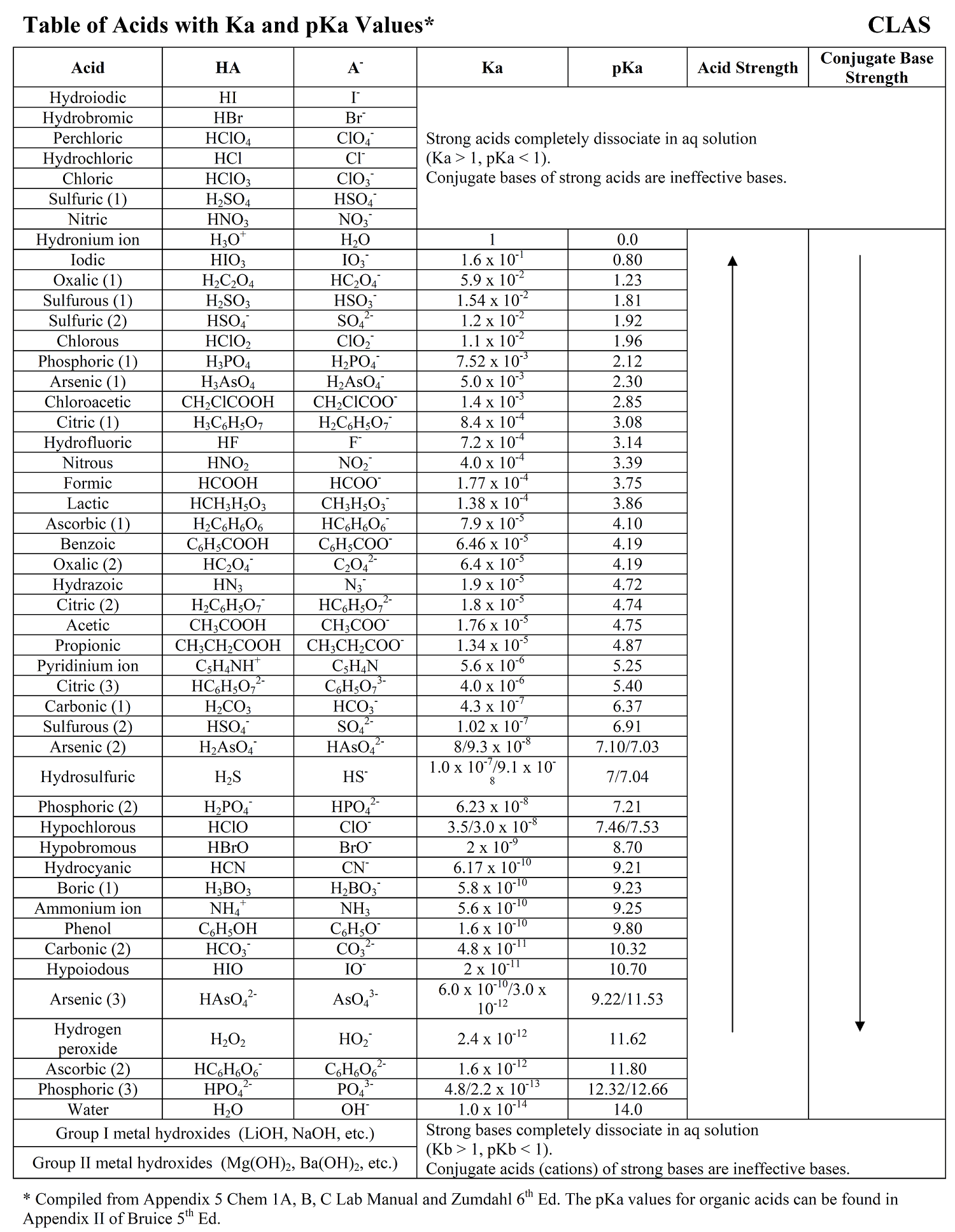## General Chemistry

Acids are classified as strong and weak depending on the extent of their ionization.

For example, hydrochloric acid is a strong acid because it completely dissociates in aqueous solutions.

HCl(aq) + H2O(l) → H3O+(aq) + Cl(aq)

or

HCl(aq) → H+(aq) + Cl(aq)

So, let’s say a bottle reads 2.0 M HCl solution. This means the concentration of hydronium and chloride ions is 2.0 M each, and there is virtually no intact HCl left in the solution as it is almost entirely ionized to ions.

On the other hand, a very small portion of nitrous acid dissociates in water and an equilibrium is established which, remember, is shown with a double arrow. Nitrous acid, therefore, is a weak acid.

HNO2(aq) + H2O(l) ⇆ H3O+(aq) + NO2(aq)

HNO2(aq) ⇆ H+(aq) + NO2(aq)

So, one measure of acid strength is the extent of its dissociation which indicates the amount of protons or hydronium ions that it produces in the solution.

The more the acid dissociates, the stronger it is. Tailoring it more towards the Brønsted–Lowry theory,  we can also say, the stronger the acid, the stronger its tendency to donate a proton.

Below are shown the most common strong acids that you need to recognize. All of them are monoprotic acids which contain only one ionizable proton. One important exception is sulfuric acid which despite being diprotic, is an example of a very strong acid.# Acid Ionization Constant (Ka)

Aside from the qualitative comparison of the acid strength, we need a quantitative definition for the acid strength. For weak acids, the dissociation is a reversible reaction described by an equilibrium constant (Keq) which, for the ionization of acids is denoted as Ka.

The dissociation of a general acid HA can be shown as:

HA(aq) + H2O(l ) ⇆ H3O+(aq) + A(aq)

The equilibrium constant for this reaction would be:

${K_{{\rm{eq}}}}\; = \,\frac{{{\rm{[}}{{\rm{H}}_{\rm{3}}}{{\rm{O}}^{\rm{ + }}}{\rm{][}}{{\rm{A}}^{\rm{ – }}}{\rm{]}}}}{{{\rm{[HA][}}{{\rm{H}}_{\rm{2}}}{\rm{O]}}}}$

Since the concentration of the water is constant and very large compared to the acid, it is included in the formula of Ka:

Ka = Keq [H2O]

Therefore, the [H2O] term does not appear in the Ka expression, and we have:

${K_{{\rm{a}}}}\; = \,\frac{{{\rm{[}}{{\rm{H}}_{\rm{3}}}{{\rm{O}}^{\rm{ + }}}{\rm{][}}{{\rm{A}}^{\rm{ – }}}{\rm{]}}}}{{{\rm{[HA]}}}}$

Just like in any equilibrium reaction, the stronger the acid, the larger the Ka because the nominator becomes greater. The weaker the acid, the less it ionizes and therefore, the smaller the ionization constant.

Whether stronger or weaker, the ionization constants for all weak acids are relatively small, but they still vary in magnitude. The values of acid dissociation constants for the most common inorganic acids are listed in the table below:# Acid Strength and pKa

Notice that all Ka values are fairly small which makes it inconvenient for certain calculations or quickly tell which acid is stronger or weaker. To simplify the numbers, the negative logarithm of Ka is often used to get rid of the exponent. This is what we also saw when introducing the pH to quantify the acidity of the solution.

pKa = – log Ka

For example, the pKa of hydrochloric, nitrous, and acetic acids are:

pKa (HCl) = – log 1.3 x 106  = -6

pKa (HNO2) = – log 7.2 x 10-4 = 3.1

pKa (CH3CO2H) = – log 1.7 x 10-5 = 4.76

Based on the Ka values, HCl is the strongest acid followed by HNO2, and then CH3CO2H. HCl has the smallest pKa, while the weakest among these, the acetic acid has the largest pKa value.

So, remember this trait – stronger acids have lower pKa.Notice also that the pKa scale is logarithmic, so even a small difference, let’s say pKa1 = 16 and pKa2 = 19 represents 3 pKa units, which means the first compound is 1,000 times more acidic than the other.

The pKa values of most common acids are given next to the corresponding Ka values in the table we have shown above.

# The Correlation of Acid and Base Strengths

So far, we have talked about the strength of the acid. However, it is also very important to understand the correlation between the strength of an acid and its conjugate base. Let’s look at the dissociation of hypochlorous and acetic acids:

HClO(aq)  ⇆ H+(aq) + ClO(aq) Ka (HClO) = 3.8 x 10-8

CH3CO2H(aq)  ⇆ H+(aq) + CH3CO2(aqKa (CH3CO2H) = 1.7 x 10-5

The Ka values indicate that acetic acid is about 1,000 times stronger than hypochlorous acid, and the equilibrium is shifted more on the right side (more dissociation) compared to that of hypochlorous acid. To signify the role of the conjugate base, we can say that the acetate ion (CH3CO2) is not as reactive as the ClO ion because otherwise the equilibrium of the second reaction would have been shifted to the left as much as it is for the dissociation of HClO. The hypochlorite ion is more reactive towards the proton while the acetate is more stable – less reactive/active otherwise it would have reacted with the proton as much as the ClO does. All of this, in one word, means that the ClO is a stronger base than the CH3CO2. So, it turns out that the stronger acid CH3CO2H has a weaker conjugate base – CH3CO2is a weaker base than ClO.

And this correlation is true for any acid and conjugate base pair:Summarizing the key patterns of the acid strength, remember that:

• The larger the Ka, the stronger the acid.
• The smaller the pKa, the stronger the acid.
• The stronger the acid, the weaker its conjugate base.
• The weaker the acid, the stronger its conjugate base.

Check Also